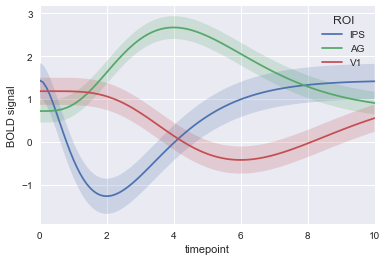This is an experiment to see if I can get a jupyter notebook to play nicely with Cryogen. It looks like it works! The workflow is to use nbconvert to turn the notebook to markdown, add the required cryogen header map, and then inline the images as base64.

The formatting is far from pretty, alas.

Here's the quick one-off script I used to inline the images from the nbconvert-generated markdown. Everything below that script came from a jupyter notebook!

import re

def makeb64img(filename):
with open(filename, "rb") as ju:
return data.encode("base64").replace('\n', '')

def swapout(match):
newstring = "data:image/png;base64," + makeb64img(match.group(2))
return match.group(1) + newstring + match.group(3)

with open("jupyter-experiment.md") as je:

regex = r"(!$png$$$)(.*png)($$)"

newpage = re.sub(regex, swapout, post)

with open("jupyter-experiment-with-image.md", "w") as je2:
je2.write(newpage)


The content of the original notebook follows:

print("here's some code!")

here's some code!
# and here's an image, stolen from seaborn examples at https://seaborn.pydata.org/examples/timeseries_from_dataframe.html
import warnings
warnings.simplefilter(action='ignore', category=UserWarning)
%matplotlib inline
import seaborn as sns
sns.set(style="darkgrid")


<matplotlib.axes._subplots.AxesSubplot at 0x111ecae48>